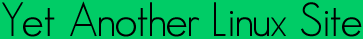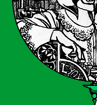Quick ?s
Cheat Sheets
Man Pages
The Lynx
Software
 ```DC(1) DC(1) NAME dc - an arbitrary precision calculator SYNOPSIS dc [-V] [--version] [-h] [--help] [-e scriptexpression] [--expression=scriptexpression] [-E scriptexpression] [--initexpr=scriptexpression] [-f scriptfile] [--file=scriptfile] [file ...] DESCRIPTION Dc is a reverse-polish desk calculator which supports unlimited preci sion arithmetic. It also allows you to define and call macros. Nor mally dc reads from the standard input; if any command arguments are given to it, they are filenames, and dc reads and executes the contents of the files before reading from standard input. All normal output is to standard output; all error output is to standard error. A reverse-polish calculator stores numbers on a stack. Entering a num ber pushes it on the stack. Arithmetic operations pop arguments off the stack and push the results. To enter a number in dc, type the digits with an optional decimal point. Exponential notation is not supported. To enter a negative number, begin the number with _. - cannot be used for this, as it is a binary operator for subtraction instead. To enter two numbers in succession, separate them with spaces or newlines. These have no meaning as commands. OPTIONS Dc may be invoked with the following command-line options: -V --version Print out the version of dc that is being run and a copyright notice, then exit. -h --help Print a usage message briefly summarizing these command-line options and the bug-reporting address, then exit. -e script --expression=script Add the commands in script to the set of commands to be run while processing the input. -E script --initexpr=script Add the commands in script to the set of commands to be run while processing the input, but unlike -e, execute stdin if no other files specified. -f script-file --file=script-file Add the commands contained in the file script-file to the set of commands to be run while processing the input. If any command-line parameters remain after processing the above, these parameters are interpreted as the names of input files to be processed. A file name of - refers to the standard input stream. The standard input will processed if no script files or expressions are specified. Printing Commands p Prints the value on the top of the stack, without altering the stack. A newline is printed after the value. n Prints the value on the top of the stack, popping it off, and does not print a newline after. P Pops off the value on top of the stack. If it it a string, it is simply printed without a trailing newline. Otherwise it is a number, and the integer portion of its absolute value is printed out as a "base (UCHAR_MAX+1)" byte stream. Assuming that (UCHAR_MAX+1) is 256 (as it is on most machines with 8-bit bytes), the sequence KSK0k1/_1Ss [ls*]Sxd0>x [256~Ssd0qaPlxx] dsxxsx0sqLqsxLxLK+k could also accomplish this function. (Much of the complexity of the above native-dc code is due to the ~ computing the characters backwards, and the desire to ensure that all registers wind up back in their original states.) f Prints the entire contents of the stack without altering any thing. This is a good command to use if you are lost or want to figure out what the effect of some command has been. Arithmetic + Pops two values off the stack, adds them, and pushes the result. The precision of the result is determined only by the values of the arguments, and is enough to be exact. - Pops two values, subtracts the first one popped from the second one popped, and pushes the result. * Pops two values, multiplies them, and pushes the result. The number of fraction digits in the result depends on the current precision value and the number of fraction digits in the two arguments. / Pops two values, divides the second one popped from the first one popped, and pushes the result. The number of fraction dig its is specified by the precision value. % Pops two values, computes the remainder of the division that the / command would do, and pushes that. The value computed is the same as that computed by the sequence Sd dld/ Ld*- . ~ Pops two values, divides the second one popped from the first one popped. The quotient is pushed first, and the remainder is pushed next. The number of fraction digits used in the division is specified by the precision value. (The sequence SdSn lnld/ LnLd% could also accomplish this function, with slightly differ ent error checking.) ^ Pops two values and exponentiates, using the first value popped as the exponent and the second popped as the base. The fraction part of the exponent is ignored. The precision value specifies the number of fraction digits in the result. | Pops three values and computes a modular exponentiation. The first value popped is used as the reduction modulus; this value must be a non-zero number, and should be an integer. The second popped is used as the exponent; this value must be a non-nega tive number, and any fractional part of this exponent will be ignored. The third value popped is the base which gets exponen tiated, which should be an integer. For small integers this is like the sequence Sm^Lm%, but, unlike ^, this command will work with arbitrarily large exponents. v Pops one value, computes its square root, and pushes that. The precision value specifies the number of fraction digits in the result. Most arithmetic operations are affected by the precision value, which you can set with the k command. The default precision value is zero, which means that all arithmetic except for addition and subtrac tion produces integer results. Stack Control c Clears the stack, rendering it empty. d Duplicates the value on the top of the stack, pushing another copy of it. Thus, 4d*p computes 4 squared and prints it. r Reverses the order of (swaps) the top two values on the stack. (This can also be accomplished with the sequence SaSbLaLb.) Registers Dc provides at least 256 memory registers, each named by a single char acter. You can store a number or a string in a register and retrieve it later. sr Pop the value off the top of the stack and store it into regis ter r. lr Copy the value in register r and push it onto the stack. This does not alter the contents of r. Each register also contains its own stack. The current register value is the top of the registers stack. Sr Pop the value off the top of the (main) stack and push it onto the stack of register r. The previous value of the register becomes inaccessible. Lr Pop the value off the top of register rs stack and push it onto the main stack. The previous value in register rs stack, if any, is now accessible via the lr command. Parameters Dc has three parameters that control its operation: the precision, the input radix, and the output radix. The precision specifies the number of fraction digits to keep in the result of most arithmetic operations. The input radix controls the interpretation of numbers typed in; all numbers typed in use this radix. The output radix is used for printing numbers. The input and output radices are separate parameters; you can make them unequal, which can be useful or confusing. The input radix must be between 2 and 16 inclusive. The output radix must be at least 2. The precision must be zero or greater. The precision is always measured in decimal digits, regardless of the current input or output radix. i Pops the value off the top of the stack and uses it to set the input radix. o Pops the value off the top of the stack and uses it to set the output radix. k Pops the value off the top of the stack and uses it to set the precision. I Pushes the current input radix on the stack. O Pushes the current output radix on the stack. K Pushes the current precision on the stack. Strings Dc has a limited ability to operate on strings as well as on numbers; the only things you can do with strings are print them and execute them as macros (which means that the contents of the string are processed as dc commands). All registers and the stack can hold strings, and dc always knows whether any given object is a string or a number. Some commands such as arithmetic operations demand numbers as arguments and print errors if given strings. Other commands can accept either a num ber or a string; for example, the p command can accept either and prints the object according to its type. [characters] Makes a string containing characters (contained between balanced [ and ] characters), and pushes it on the stack. For example, [foo]P prints the characters foo (with no newline). a The top-of-stack is popped. If it was a number, then the low- order byte of this number is converted into a string and pushed onto the stack. Otherwise the top-of-stack was a string, and the first character of that string is pushed back. x Pops a value off the stack and executes it as a macro. Normally it should be a string; if it is a number, it is simply pushed back onto the stack. For example, [1p]x executes the macro 1p which pushes 1 on the stack and prints 1 on a separate line. Macros are most often stored in registers; [1p]sa stores a macro to print 1 into register a, and lax invokes this macro. >r Pops two values off the stack and compares them assuming they are numbers, executing the contents of register r as a macro if the original top-of-stack is greater. Thus, 1 2>a will invoke register as contents and 2 1>a will not. !>r Similar but invokes the macro if the original top-of-stack is not greater than (less than or equal to) what was the second-to- top. commands take precedence, so if you want to run a command starting with <, =, or > you will need to add a space after the !. # Will interpret the rest of the line as a comment. :r Will pop the top two values off of the stack. The old second- to-top value will be stored in the array r, indexed by the old top-of-stack value. ;r Pops the top-of-stack and uses it as an index into the array r. The selected value is then pushed onto the stack. Note that each stacked instance of a register has its own array associ ated with it. Thus 1 0:a 0Sa 2 0:a La 0;ap will print 1, because the 2 was stored in an instance of 0:a that was later popped. FILES ~/.dcrc The commands in this file will be executed when dc is first run. BUGS Email bug reports to bug-dc@gnu.org. GNU Project 2001-05-13 DC(1) ```Yals.net is © 1999-2009 Crescendo Communications Sharing tech info on the web for more than a decade! This page was generated Thu Apr 30 17:05:18 2009